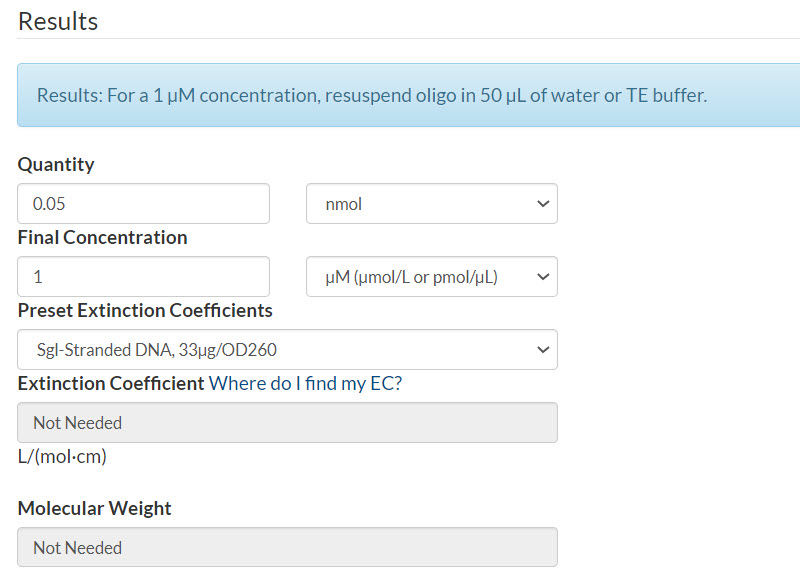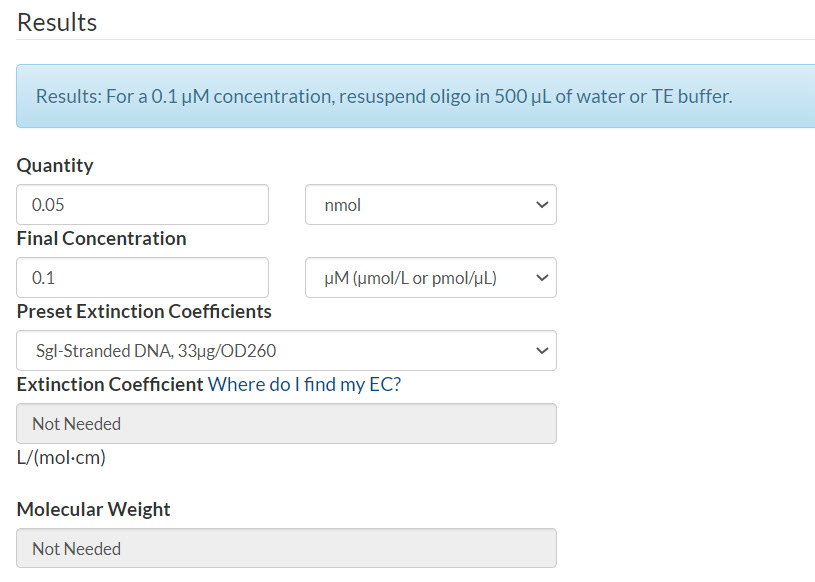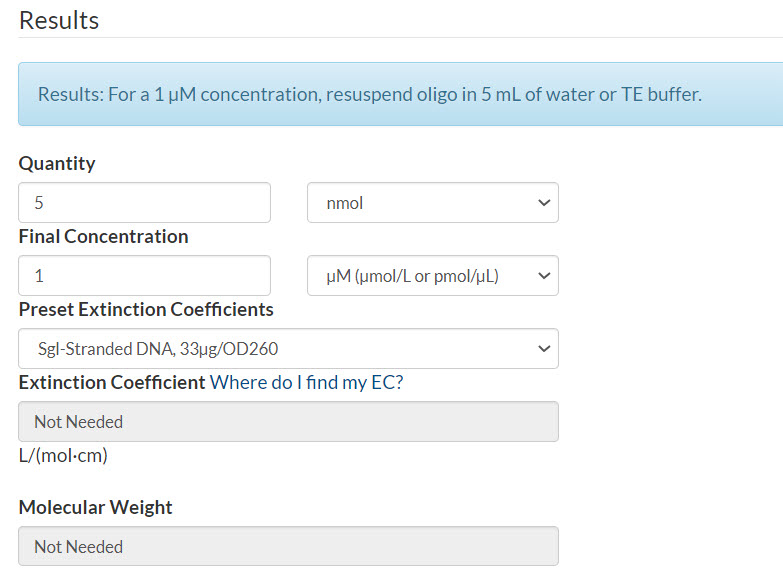Our Scientific Applications Support team has assembled a list of frequently asked questions to help you find answers quickly. Filter using one or more categories to focus on specific topics, or use the search bar to perform a text search.

# How do I resuspend my oPools™ Oligo Pools to my desired concentration?

Calculate the appropriate resuspension volume for your oPools product with the  IDT Resuspension Calculator.

First, convert the oPool scale—provided in pmol—to a nmol quantity.

If your desired final concentration is µM per oligo, use this equation to determine your quantity:

pmol per oligo / 1000 = # nmol per oligo

Example 1. Final concentration of 1 µM per oligo.
Scenario: 50 pmol oPools Oligo Pool
Quantity: 50 pmol oPools Oligo Pool / 1000 = 0.05 nmol
Final concentration: 1 µM
Resuspension calculator (Figure 1):

• Enter the quantity that you calculated
• Select Sgl-Stranded DNA (single-stranded DNA)
• Click CalculateFigure 1. 50 pmol oPools Oligo Pool resuspended to 1 µM per oligo.

If your desired final concentration is nM per oligo, you can convert using this equation:

nM concentration / 1000 = # µM

Example 2. Final concentration of 100 nM per oligo.
Scenario: 50 pmol oPools Oligo Pool
Quantity: 50 pmol oPools Oligo Pool / 1000 = 0.05 nmol
Final concentration in µM: 100 nM  / 1000 = 0.1 µM
Resuspension calculator (Figure 2):

• Enter the quantity that you calculated
• Enter your final concentration per oligo that you calculated
• Select Sgl-Stranded DNA (single-stranded DNA)
• Click CalculateFigure 2. 50 pmol oPools Oligo Pool resuspended to 100 nM per oligo.

If your desired final concentration is per pool, use this equation:

(pmol per oligo x # of oligos in pool) / 1000 = # nmol per pool

Example 3. Final concentration of 1 µM per pool.
Scenario: 50 pmol oPools Oligo Pool containing 100 oligos
Quantity: (50 pmol x 100 oligos) / 1000 = 5 nmol
Final concentration: 1 µM
Resuspension calculator (Figure 3):

• Enter the quantity that you calculated